### NumPy Ndarray 对象

NumPy 最重要的一个特点是其 N 维数组对象 ndarray，它是一系列同类型数据的集合，以 0 下标为开始进行集合中元素的索引。

ndarray 对象是用于存放同类型元素的多维数组。

ndarray 中的每个元素在内存中都有相同存储大小的区域。

ndarray 内部由以下内容组成：

• 一个指向数据（内存或内存映射文件中的一块数据）的指针。

• 数据类型或 dtype，描述在数组中的固定大小值的格子。

• 一个表示数组形状（shape）的元组，表示各维度大小的元组。

• 一个跨度元组（stride），其中的整数指的是为了前进到当前维度下一个元素需要”跨过”的字节数。

ndarray 的内部结构: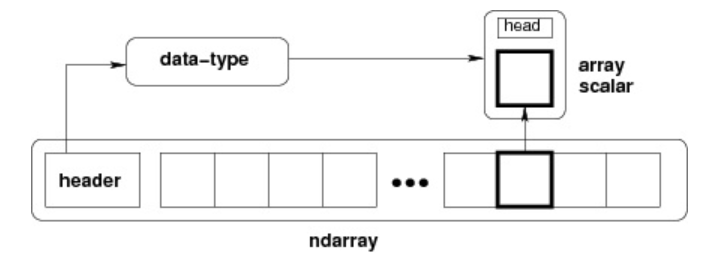``````numpy.array(object, dtype = None, copy = True, order = None, subok = False, ndmin = 0)
``````
• 1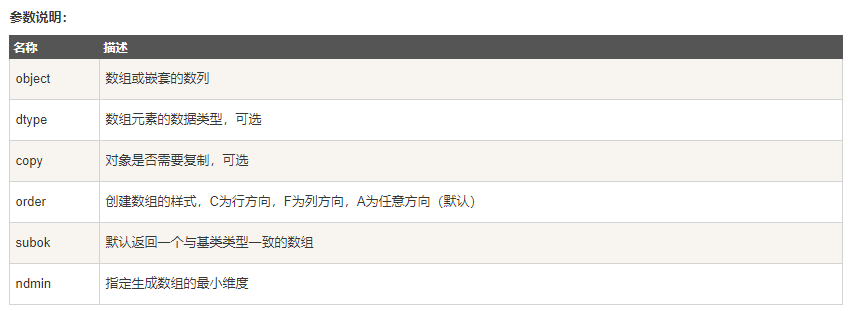### NumPy 数据类型

numpy 支持的数据类型比 Python 内置的类型要多很多，基本上可以和 C 语言的数据类型对应上，其中部分类型对应为 Python 内置的类型。下表列举了常用 NumPy 基本类型。

• bool_ 布尔型数据类型（True 或者 False）
• int_ 默认的整数类型（类似于 C 语言中的 long，int32 或 int64）
• intc 与 C 的 int 类型一样，一般是 int32 或 int 64
• intp 用于索引的整数类型（类似于 C 的 ssize_t，一般情况下仍然是 int32 或 int64）
• int8 字节（-128 to 127）
• int16 整数（-32768 to 32767）
• int32 整数（-2147483648 to 2147483647）
• int64 整数（-9223372036854775808 to 9223372036854775807）
• uint8 无符号整数（0 to 255）
• uint16 无符号整数（0 to 65535）
• uint32 无符号整数（0 to 4294967295）
• uint64 无符号整数（0 to 18446744073709551615）
• float_ float64 类型的简写
• float16 半精度浮点数，包括：1 个符号位，5 个指数位，10 个尾数位
• float32 单精度浮点数，包括：1 个符号位，8 个指数位，23 个尾数位
• float64 双精度浮点数，包括：1 个符号位，11 个指数位，52 个尾数位
• complex_ complex128 类型的简写，即 128 位复数
• complex64 复数，表示双 32 位浮点数（实数部分和虚数部分）
• complex128 复数，表示双 64 位浮点数（实数部分和虚数部分）

numpy 的数值类型实际上是 dtype 对象的实例，并对应唯一的字符，包括 np.bool_，np.int32，np.float32，等等。

### 数据类型对象 (dtype)

• 数据的类型（整数，浮点数或者 Python 对象）
• 数据的大小（例如， 整数使用多少个字节存储）
• 数据的字节顺序（小端法或大端法）
• 在结构化类型的情况下，字段的名称、每个字段的数据类型和每个字段所取的内存块的部分
• 如果数据类型是子数组，它的形状和数据类型
字节顺序是通过对数据类型预先设定”<“或”>“来决定的。”<“意味着小端法(最小值存储在最小的地址，即低位组放在最前面)。”>”意味着大端法(最重要的字节存储在最小的地址，即高位组放在最前面)。

dtype 对象是使用以下语法构造的：

``````numpy.dtype(object, align, copy)
``````
• 1
• object – 要转换为的数据类型对象
• align – 如果为 true，填充字段使其类似 C 的结构体。
• copy – 复制 dtype 对象 ，如果为 false，则是对内置数据类型对象的引用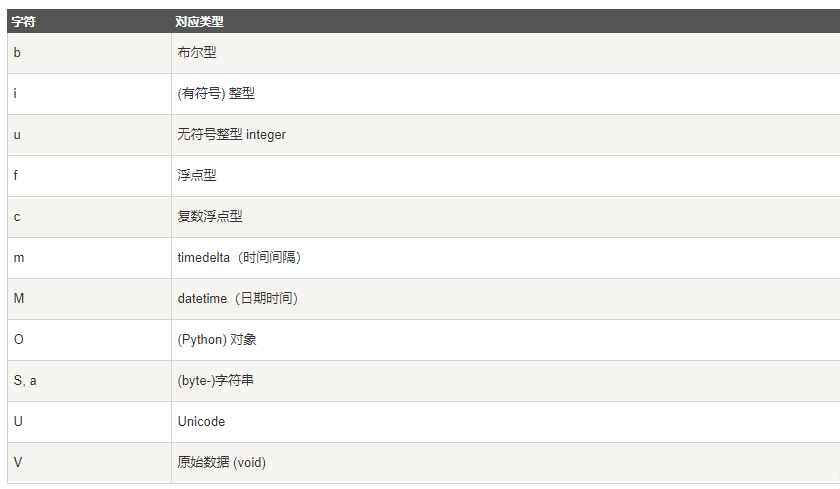### NumPy 数组属性

NumPy 数组的维数称为秩（rank），一维数组的秩为 1，二维数组的秩为 2，以此类推。

NumPy 的数组中比较重要 ndarray 对象属性有：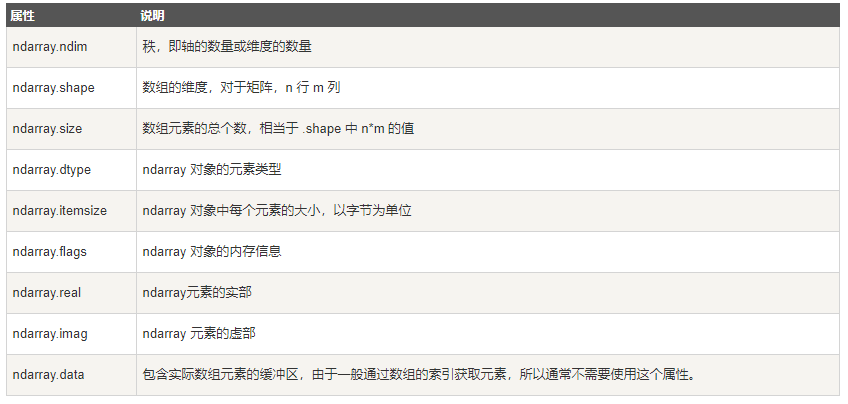ndarray.flags 返回 ndarray 对象的内存信息，包含以下属性：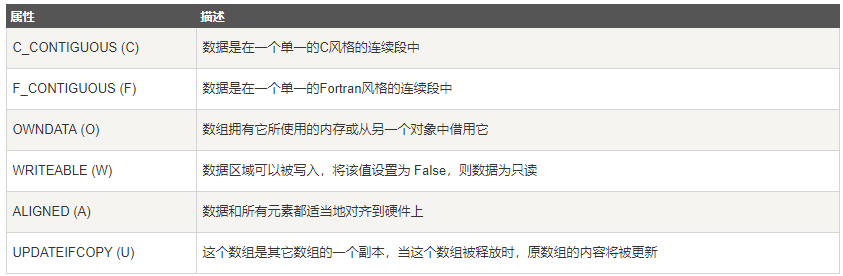### NumPy 创建数组

ndarray 数组除了可以使用底层 ndarray 构造器来创建外，也可以通过以下几种方式来创建。

numpy.empty

numpy.empty 方法用来创建一个指定形状（shape）、数据类型（dtype）且未初始化的数组：

``````numpy.empty(shape, dtype = float, order = 'C')
``````
• 1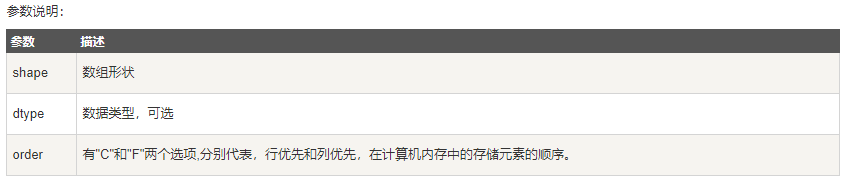numpy.zeros

``````numpy.zeros(shape, dtype = float, order = 'C')
``````
• 1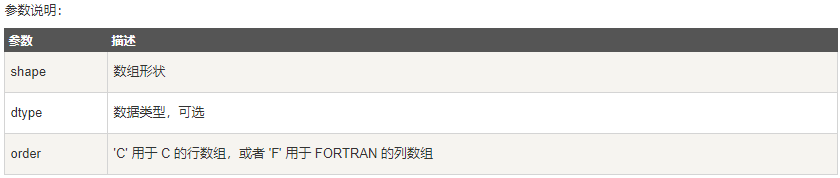numpy.ones

``````numpy.ones(shape, dtype = None, order = 'C')
``````
• 1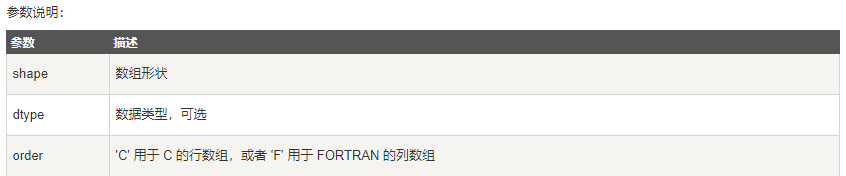### NumPy 从已有的数组创建数组

numpy.asarray
numpy.asarray 类似 numpy.array，但 numpy.asarray 只有三个，比 numpy.array 少两个。

``````numpy.asarray(a, dtype = None, order = None)
``````
• 1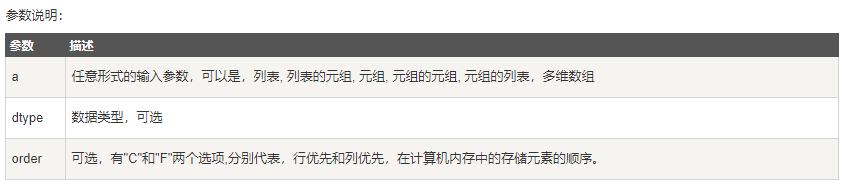numpy.frombuffer
numpy.frombuffer 用于实现动态数组。

numpy.frombuffer 接受 buffer 输入参数，以流的形式读入转化成 ndarray 对象。

``````numpy.frombuffer(buffer, dtype = float, count = -1, offset = 0)
``````
• 1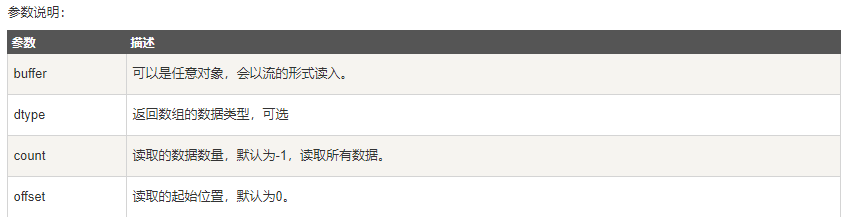numpy.fromiter
numpy.fromiter 方法从可迭代对象中建立 ndarray 对象，返回一维数组。

``````numpy.fromiter(iterable, dtype, count=-1)
``````
• 1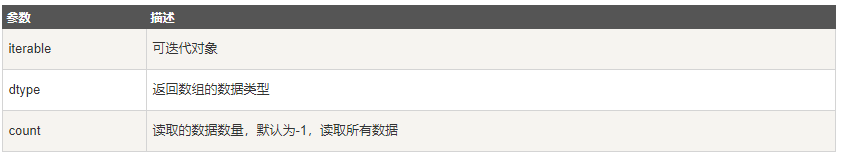### NumPy 从数值范围创建数组

numpy.arange
numpy 包中的使用 arange 函数创建数值范围并返回 ndarray 对象，函数格式如下：

``````numpy.arange(start, stop, step, dtype)
``````
• 1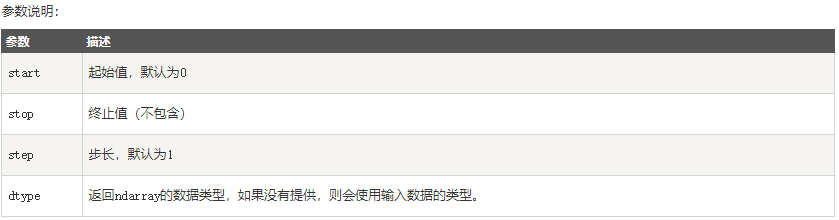numpy.linspace
numpy.linspace 函数用于创建一个一维数组，数组是一个等差数列构成的，格式如下：

``````np.linspace(start, stop, num=50, endpoint=True, retstep=False, dtype=None)
``````
• 1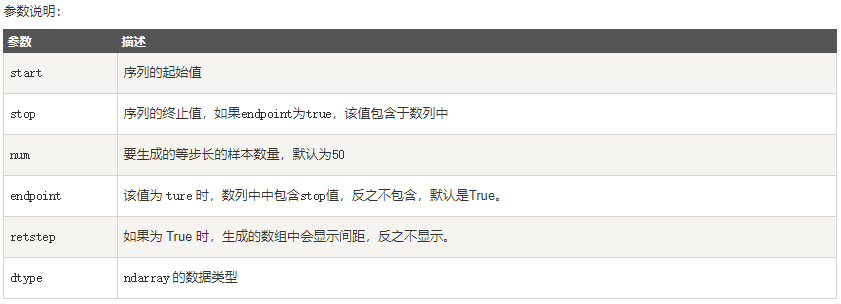numpy.logspace
numpy.logspace 函数用于创建一个于等比数列。格式如下：

``````np.logspace(start, stop, num=50, endpoint=True, base=10.0, dtype=None)
``````
• 1

base 参数意思是取对数的时候 log 的下标。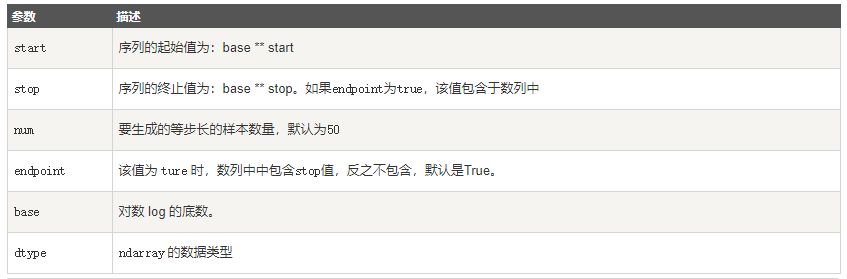### NumPy 切片和索引

ndarray对象的内容可以通过索引或切片来访问和修改，与 Python 中 list 的切片操作一样。

ndarray 数组可以基于 0 – n 的下标进行索引，切片对象可以通过内置的 slice 函数，并设置 start, stop 及 step 参数进行，从原数组中切割出一个新数组。

``````import numpy as np

a = np.arange(10)
s = slice(2,7,2)   # 从索引 2 开始到索引 7 停止，间隔为2
print (a[s])
``````
• 1
• 2
• 3
• 4
• 5

``````[2  4  6]
``````
• 1

``````import numpy as np

a = np.arange(10)
b = a[2:7:2]   # 从索引 2 开始到索引 7 停止，间隔为 2
print(b)
``````
• 1
• 2
• 3
• 4
• 5

``````[2  4  6]
``````
• 1

``````import numpy as np

a = np.arange(10)  # [0 1 2 3 4 5 6 7 8 9]
b = a
print(b)
``````
• 1
• 2
• 3
• 4
• 5

``````5
``````
• 1

``````import numpy as np

a = np.arange(10)
print(a[2:])
``````
• 1
• 2
• 3
• 4

``````[2  3  4  5  6  7  8  9]
``````
• 1

``````import numpy as np

a = np.arange(10)  # [0 1 2 3 4 5 6 7 8 9]
print(a[2:5])
``````
• 1
• 2
• 3
• 4

``````[2  3  4]
``````
• 1

``````import numpy as np

a = np.array([[1,2,3],[3,4,5],[4,5,6]])
print(a)
# 从某个索引处开始切割
print('从数组索引 a[1:] 处开始切割')
print(a[1:])
``````
• 1
• 2
• 3
• 4
• 5
• 6
• 7

``````[[1 2 3]
[3 4 5]
[4 5 6]]
``````
• 1
• 2
• 3

``````[[3 4 5]
[4 5 6]]
``````
• 1
• 2

``````import numpy as np

a = np.array([[1,2,3],[3,4,5],[4,5,6]])
print (a[...,1])   # 第2列元素
print (a[1,...])   # 第2行元素
print (a[...,1:])  # 第2列及剩下的所有元素
``````
• 1
• 2
• 3
• 4
• 5
• 6

``````[2 4 5]
[3 4 5]
[[2 3]
[4 5]
[5 6]]
``````
• 1
• 2
• 3
• 4
• 5

### NumPy 高级索引

• 整数数组索引
• 布尔索引
• 花式索引

``````import numpy as np

a = np.array([1,2,3,4])
b = np.array([10,20,30,40])
c = a * b
print (c)
``````
• 1
• 2
• 3
• 4
• 5
• 6

``````[ 10  40  90 160]
``````
• 1

``````import numpy as np

a = np.array([[ 0, 0, 0],
[10,10,10],
[20,20,20],
[30,30,30]])
b = np.array([1,2,3])
print(a + b)
``````
• 1
• 2
• 3
• 4
• 5
• 6
• 7
• 8

``````[[ 1  2  3]
[11 12 13]
[21 22 23]
[31 32 33]]
``````
• 1
• 2
• 3
• 4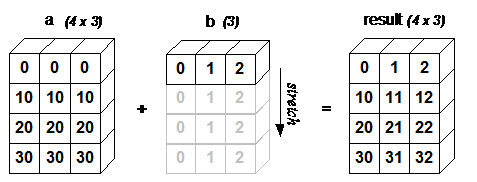• 让所有输入数组都向其中形状最长的数组看齐，形状中不足的部分都通过在前面加 1 补齐。
• 输出数组的形状是输入数组形状的各个维度上的最大值。
• 如果输入数组的某个维度和输出数组的对应维度的长度相同或者其长度为 1 时，这个数组能够用来计算，否则出错。
• 当输入数组的某个维度的长度为 1 时，沿着此维度运算时都用此维度上的第一组值。

• 数组拥有相同形状。
• 当前维度的值相等。
• 当前维度的值有一个是 1。

### NumPy 迭代数组

NumPy 迭代器对象 numpy.nditer 提供了一种灵活访问一个或者多个数组元素的方式。

``````import numpy as np

a = np.arange(6).reshape(2,3)
print ('原始数组是：')
print (a)
print ('\n')
print ('迭代输出元素：')
for x in np.nditer(a):
print (x, end=", " )
print ('\n')
``````
• 1
• 2
• 3
• 4
• 5
• 6
• 7
• 8
• 9
• 10

``````原始数组是：
[[0 1 2]
[3 4 5]]

0, 1, 2, 3, 4, 5,
``````
• 1
• 2
• 3
• 4
• 5
• 6
• 7

``````import numpy as np

a = np.arange(6).reshape(2,3)
for x in np.nditer(a.T):
print (x, end=", " )
print ('\n')

for x in np.nditer(a.T.copy(order='C')):
print (x, end=", " )
print ('\n')
``````
• 1
• 2
• 3
• 4
• 5
• 6
• 7
• 8
• 9
• 10

``````0, 1, 2, 3, 4, 5,

0, 3, 1, 4, 2, 5,
``````
• 1
• 2
• 3

for x in np.nditer(a, order=‘F’):Fortran order，即是列序优先；
for x in np.nditer(a.T, order=‘C’):C order，即是行序优先；

nditer类的构造器拥有flags参数，它可以接受下列值：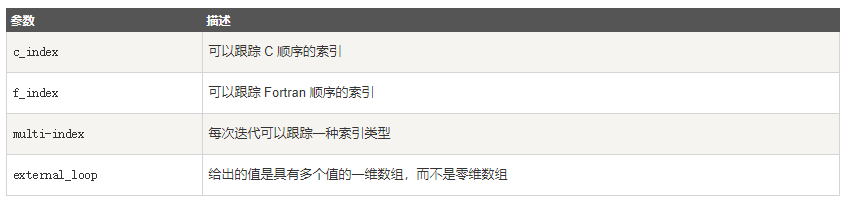### Numpy 数组操作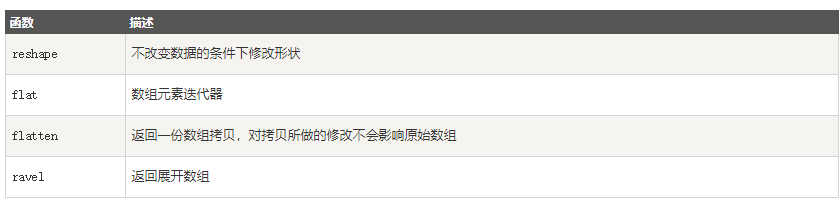numpy.reshape
numpy.reshape 函数可以在不改变数据的条件下修改形状，格式如下： numpy.reshape(arr, newshape, order=‘C’)

• arr：要修改形状的数组
• newshape：整数或者整数数组，新的形状应当兼容原有形状
• order：‘C’ – 按行，‘F’ – 按列，‘A’ – 原顺序，‘k’ – 元素在内存中的出现顺序。

numpy.ndarray.flat
numpy.ndarray.flat 是一个数组元素迭代器

numpy.ndarray.flatten
numpy.ndarray.flatten 返回一份数组拷贝，对拷贝所做的修改不会影响原始数组，格式如下：

``````ndarray.flatten(order='C')
``````
• 1

• order：‘C’ – 按行，‘F’ – 按列，‘A’ – 原顺序，‘K’ – 元素在内存中的出现顺序。

numpy.ravel
numpy.ravel() 展平的数组元素，顺序通常是”C风格”，返回的是数组视图（view，有点类似 C/C++引用reference的意味），修改会影响原始数组。

``````numpy.ravel(a, order='C')
``````
• 1

• order：‘C’ – 按行，‘F’ – 按列，‘A’ – 原顺序，‘K’ – 元素在内存中的出现顺序。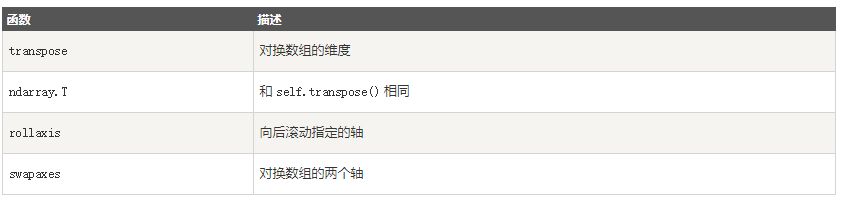numpy.transpose
numpy.transpose 函数用于对换数组的维度，格式如下：

``````numpy.transpose(arr, axes)
``````
• 1

• arr：要操作的数组
• axes：整数列表，对应维度，通常所有维度都会对换。

numpy.rollaxis
numpy.rollaxis 函数向后滚动特定的轴到一个特定位置，格式如下：

``````numpy.rollaxis(arr, axis, start)
``````
• 1

• arr：数组
• axis：要向后滚动的轴，其它轴的相对位置不会改变
• start：默认为零，表示完整的滚动。会滚动到特定位置。

numpy.swapaxes
numpy.swapaxes 函数用于交换数组的两个轴，格式如下：

``````numpy.swapaxes(arr, axis1, axis2)
``````
• 1
• arr：输入的数组
• axis1：对应第一个轴的整数
• axis2：对应第二个轴的整数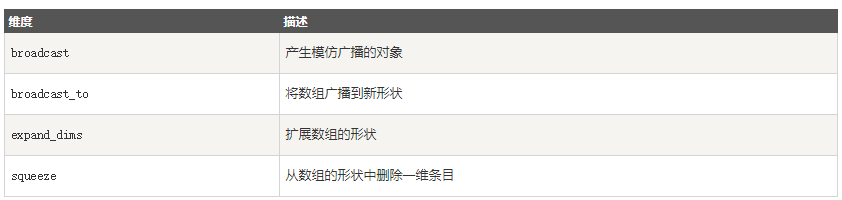numpy.broadcast_to 函数将数组广播到新形状。它在原始数组上返回只读视图。 它通常不连续。 如果新形状不符合 NumPy 的广播规则，该函数可能会抛出ValueError。

``````numpy.broadcast_to(array, shape, subok)
``````
• 1

numpy.expand_dims
numpy.expand_dims 函数通过在指定位置插入新的轴来扩展数组形状，函数格式如下:

`````` numpy.expand_dims(arr, axis)
``````
• 1

• arr：输入数组
• axis：新轴插入的位置

numpy.squeeze
numpy.squeeze 函数从给定数组的形状中删除一维的条目，函数格式如下：

``````numpy.squeeze(arr, axis)
``````
• 1

• arr：输入数组
• axis：整数或整数元组，用于选择形状中一维条目的子集

### 连接数组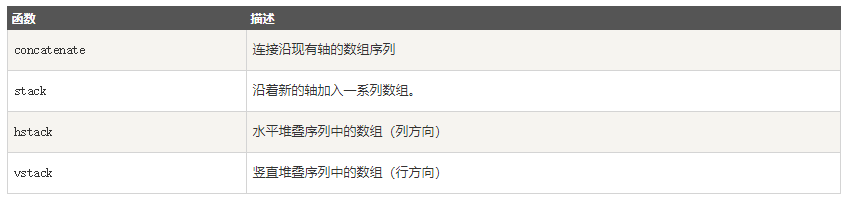numpy.concatenate
numpy.concatenate 函数用于沿指定轴连接相同形状的两个或多个数组，格式如下：

``````numpy.concatenate((a1, a2, ...), axis)
``````
• 1

• a1, a2, …：相同类型的数组
• axis：沿着它连接数组的轴，默认为 0

numpy.stack
numpy.stack 函数用于沿新轴连接数组序列，格式如下：

``````numpy.stack(arrays, axis)
``````
• 1

• arrays相同形状的数组序列
• axis：返回数组中的轴，输入数组沿着它来堆叠

numpy.hstack
numpy.hstack 是 numpy.stack 函数的变体，它通过水平堆叠来生成数组。

numpy.vstack
numpy.vstack 是 numpy.stack 函数的变体，它通过垂直堆叠来生成数组。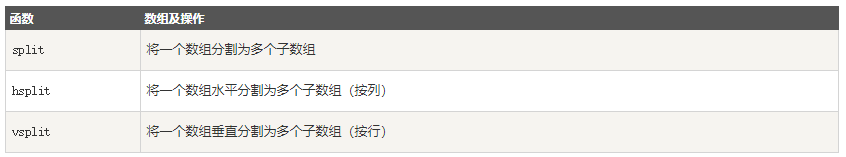numpy.split
numpy.split 函数沿特定的轴将数组分割为子数组，格式如下：

``````numpy.split(ary, indices_or_sections, axis)
``````
• 1

• ary：被分割的数组
• indices_or_sections：果是一个整数，就用该数平均切分，如果是一个数组，为沿轴切分的位置（左开右闭）
• axis：沿着哪个维度进行切向，默认为0，横向切分。为1时，纵向切分

numpy.hsplit
numpy.hsplit 函数用于水平分割数组，通过指定要返回的相同形状的数组数量来拆分原数组。

numpy.vsplit
numpy.vsplit 沿着垂直轴分割，其分割方式与hsplit用法相同。

### 数组元素的添加与删除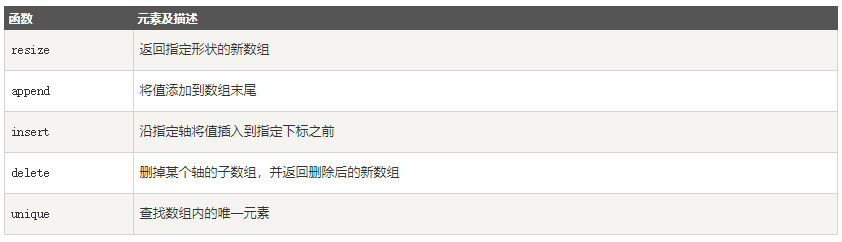numpy.resize
numpy.resize 函数返回指定大小的新数组。

``````numpy.resize(arr, shape)
``````
• 1

• arr：要修改大小的数组
• shape：返回数组的新形状

numpy.append
numpy.append 函数在数组的末尾添加值。 追加操作会分配整个数组，并把原来的数组复制到新数组中。 此外，输入数组的维度必须匹配否则将生成ValueError。

append 函数返回的始终是一个一维数组。

``````numpy.append(arr, values, axis=None)
``````
• 1

• arr：输入数组
• values：要向arr添加的值，需要和arr形状相同（除了要添加的轴）
• axis：默认为 None。当axis无定义时，是横向加成，返回总是为一维数组！当axis有定义的时候，分别为0和1的时候。当axis有定义的时候，分别为0和1的时候（列数要相同）。当axis为1时，数组是加在右边（行数要相同）。

numpy.insert
numpy.insert 函数在给定索引之前，沿给定轴在输入数组中插入值。

``````numpy.insert(arr, obj, values, axis)
``````
• 1

• arr：输入数组
• obj：在其之前插入值的索引
• values：要插入的值
• axis：沿着它插入的轴，如果未提供，则输入数组会被展开

numpy.delete
numpy.delete 函数返回从输入数组中删除指定子数组的新数组。 与 insert() 函数的情况一样，如果未提供轴参数，则输入数组将展开。

``````Numpy.delete(arr, obj, axis)
``````
• 1

• arr：输入数组
• obj：可以被切片，整数或者整数数组，表明要从输入数组删除的子数组
• axis：沿着它删除给定子数组的轴，如果未提供，则输入数组会被展开

numpy.unique
numpy.unique 函数用于去除数组中的重复元素。

``````numpy.unique(arr, return_index, return_inverse, return_counts)
``````
• 1
• arr：输入数组，如果不是一维数组则会展开
• return_index：如果为true，返回新列表元素在旧列表中的位置（下标），并以列表形式储
• return_inverse：如果为true，返回旧列表元素在新列表中的位置（下标），并以列表形式储
• return_counts：如果为true，返回去重数组中的元素在原数组中的出现次数

### NumPy 位运算

NumPy “bitwise_” 开头的函数是位运算函数。

NumPy 位运算包括以下几个函数：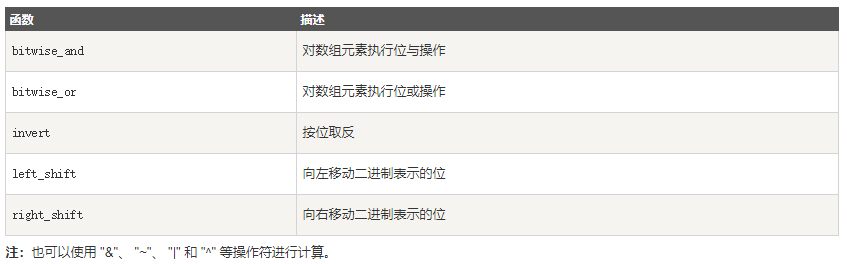### NumPy 字符串函数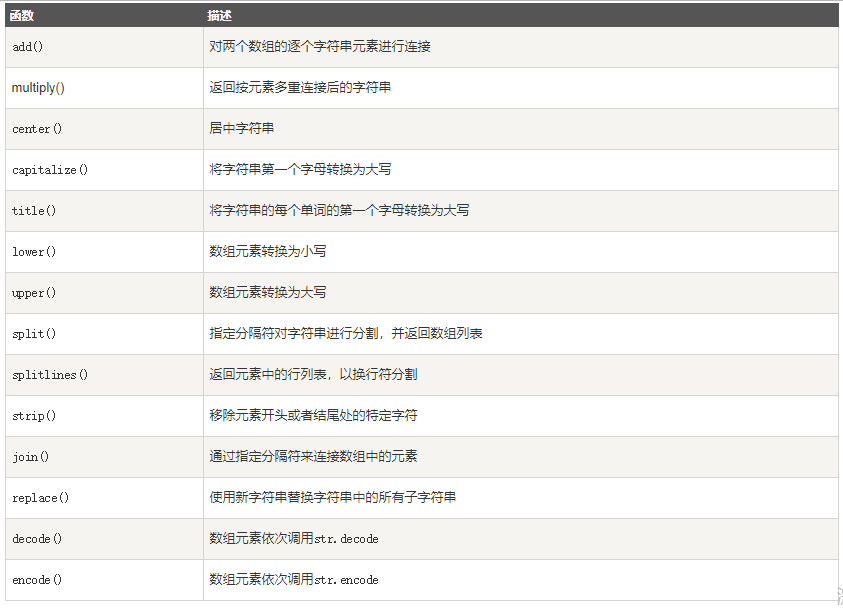### NumPy 数学函数

NumPy 提供了标准的三角函数：sin()、cos()、tan()。

numpy.around() 函数返回指定数字的四舍五入值。

``````numpy.around(a,decimals)
``````
• 1

• a: 数组
• decimals: 舍入的小数位数。 默认值为0。 如果为负，整数将四舍五入到小数点左侧的位置

numpy.floor()
numpy.floor() 返回数字的下舍整数。

numpy.ceil()
numpy.ceil() 返回数字的上入整数。

### NumPy 算术函数

numpy.reciprocal()
numpy.reciprocal() 函数返回参数逐元素的倒数。如 1/4 倒数为 4/1。

numpy.power()
numpy.power() 函数将第一个输入数组中的元素作为底数，计算它与第二个输入数组中相应元素的幂。

numpy.mod()
numpy.mod() 计算输入数组中相应元素的相除后的余数。 函数 numpy.remainder() 也产生相同的结果。

### NumPy 统计函数

numpy.amin() 和 numpy.amax()
numpy.amin() 用于计算数组中的元素沿指定轴的最小值。

numpy.amax() 用于计算数组中的元素沿指定轴的最大值。

numpy.ptp()
numpy.ptp()函数计算数组中元素最大值与最小值的差（最大值 – 最小值）。

numpy.percentile()

``````numpy.percentile(a, q, axis)
``````
• 1

• a: 输入数组
• q: 要计算的百分位数，在 0 ~ 100 之间
• axis: 沿着它计算百分位数的轴
首先明确百分位数：

numpy.median()
numpy.median() 函数用于计算数组 a 中元素的中位数（中值）

numpy.mean()
numpy.mean() 函数返回数组中元素的算术平均值。 如果提供了轴，则沿其计算。

numpy.average()
numpy.average() 函数根据在另一个数组中给出的各自的权重计算数组中元素的加权平均值。

``````std = sqrt(mean((x - x.mean())**2))
``````
• 1

### NumPy 排序、条件刷选函数

NumPy 提供了多种排序的方法。 这些排序函数实现不同的排序算法，每个排序算法的特征在于执行速度，最坏情况性能，所需的工作空间和算法的稳定性。 下表显示了三种排序算法的比较。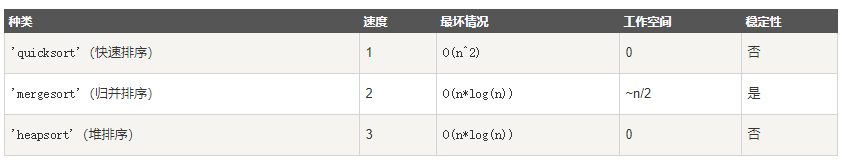numpy.sort()
numpy.sort() 函数返回输入数组的排序副本。函数格式如下：

``````numpy.sort(a, axis, kind, order)
``````
• 1

numpy.argsort()
numpy.argsort() 函数返回的是数组值从小到大的索引值。

numpy.lexsort()
numpy.lexsort() 用于对多个序列进行排序。把它想象成对电子表格进行排序，每一列代表一个序列，排序时优先照顾靠后的列。

msort、sort_complex、partition、argpartition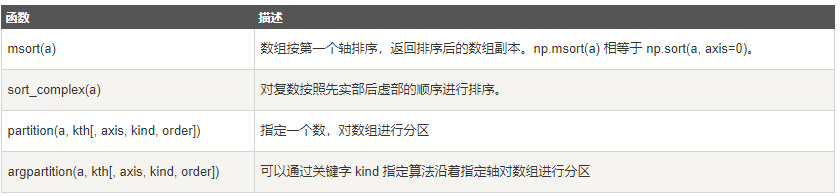numpy.argmax() 和 numpy.argmin()
numpy.argmax() 和 numpy.argmin()函数分别沿给定轴返回最大和最小元素的索引。

numpy.nonzero()
numpy.nonzero() 函数返回输入数组中非零元素的索引。

numpy.where()
numpy.where() 函数返回输入数组中满足给定条件的元素的索引。

numpy.extract()
numpy.extract() 函数根据某个条件从数组中抽取元素，返回满条件的元素。

### NumPy 字节交换

• 大端模式：指数据的高字节保存在内存的低地址中，而数据的低字节保存在内存的高地址中，这样的存储模式有点儿类似于把数据当作字符串顺序处理：地址由小向大增加，而数据从高位往低位放；这和我们的阅读习惯一致。

• 小端模式：指数据的高字节保存在内存的高地址中，而数据的低字节保存在内存的低地址中，这种存储模式将地址的高低和数据位权有效地结合起来，高地址部分权值高，低地址部分权值低。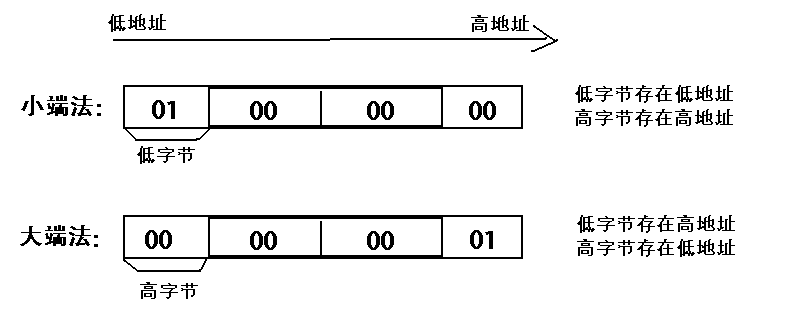numpy.ndarray.byteswap()
numpy.ndarray.byteswap() 函数将 ndarray 中每个元素中的字节进行大小端转换。

### NumPy 矩阵库(Matrix)

NumPy 中包含了一个矩阵库 numpy.matlib，该模块中的函数返回的是一个矩阵，而不是 ndarray 对象。

matlib.empty()
matlib.empty() 函数返回一个新的矩阵，语法格式为：

``````numpy.matlib.empty(shape, dtype, order)
``````
• 1

``````shape: 定义新矩阵形状的整数或整数元组
Dtype: 可选，数据类型
order: C（行序优先） 或者 F（列序优先）
``````
• 1
• 2
• 3

numpy.matlib.zeros()
numpy.matlib.zeros() 函数创建一个以 0 填充的矩阵。

numpy.matlib.ones()
numpy.matlib.ones()函数创建一个以 1 填充的矩阵。

numpy.matlib.eye()
numpy.matlib.eye() 函数返回一个矩阵，对角线元素为 1，其他位置为零。

``````numpy.matlib.eye(n, M,k, dtype)
``````
• 1

``````n: 返回矩阵的行数
M: 返回矩阵的列数，默认为 n
k: 对角线的索引
dtype: 数据类型
``````
• 1
• 2
• 3
• 4

numpy.matlib.identity()
numpy.matlib.identity() 函数返回给定大小的单位矩阵。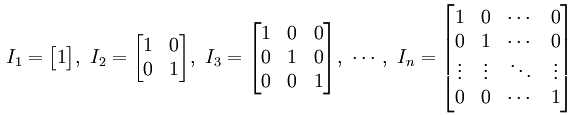numpy.matlib.rand()
numpy.matlib.rand() 函数创建一个给定大小的矩阵，数据是随机填充的。

### NumPy 线性代数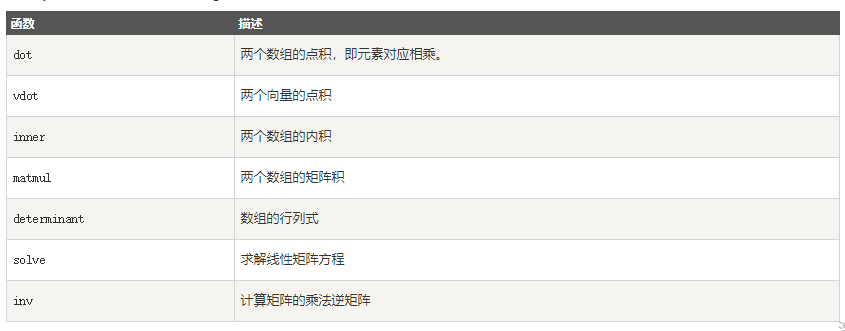numpy.dot()
numpy.dot() 对于两个一维的数组，计算的是这两个数组对应下标元素的乘积和(数学上称之为内积)；对于二维数组，计算的是两个数组的矩阵乘积；对于多维数组，它的通用计算公式如下，即结果数组中的每个元素都是：数组a的最后一维上的所有元素与数组b的倒数第二位上的所有元素的乘积和： dot(a, b)[i,j,k,m] = sum(a[i,j,:] * b[k,:,m])。

``````numpy.dot(a, b, out=None)
``````
• 1

``````a : ndarray 数组
b : ndarray 数组
out : ndarray, 可选，用来保存dot()的计算结果
``````
• 1
• 2
• 3

numpy.vdot()
numpy.vdot() 函数是两个向量的点积。 如果第一个参数是复数，那么它的共轭复数会用于计算。 如果参数是多维数组，它会被展开。

numpy.inner()
numpy.inner() 函数返回一维数组的向量内积。对于更高的维度，它返回最后一个轴上的和的乘积。

numpy.matmul
numpy.matmul 函数返回两个数组的矩阵乘积。 虽然它返回二维数组的正常乘积，但如果任一参数的维数大于2，则将其视为存在于最后两个索引的矩阵的栈，并进行相应广播。

numpy.linalg.det()
numpy.linalg.det() 函数计算输入矩阵的行列式。

numpy.linalg.solve()
numpy.linalg.solve() 函数给出了矩阵形式的线性方程的解。

x + y + z = 6

2y + 5z = -4

2x + 5y – z = 27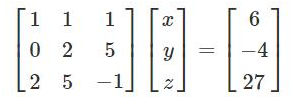AX = B

X = A^(-1)B

numpy.linalg.inv()
numpy.linalg.inv() 函数计算矩阵的乘法逆矩阵。

### NumPy IO

Numpy 可以读写磁盘上的文本数据或二进制数据。

NumPy 为 ndarray 对象引入了一个简单的文件格式：npy。

npy 文件用于存储重建 ndarray 所需的数据、图形、dtype 和其他信息。

• load() 和 save() 函数是读写文件数组数据的两个主要函数，默认情况下，数组是以未压缩的原始二进制格式保存在扩展名为 .npy 的文件中。
• savze() 函数用于将多个数组写入文件，默认情况下，数组是以未压缩的原始二进制格式保存在扩展名为 .npz 的文件中。
• loadtxt() 和 savetxt() 函数处理正常的文本文件(.txt 等)

numpy.save()
numpy.save() 函数将数组保存到以 .npy 为扩展名的文件中。

``````numpy.save(file, arr, allow_pickle=True, fix_imports=True)
``````
• 1

``````file：要保存的文件，扩展名为 .npy，如果文件路径末尾没有扩展名 .npy，该扩展名会被自动加上。
arr: 要保存的数组
allow_pickle: 可选，布尔值，允许使用 Python pickles 保存对象数组，Python 中的 pickle 用于在保存到磁盘文件或从磁盘文件读取之前，对对象进行序列化和反序列化。
fix_imports: 可选，为了方便 Pyhton2 中读取 Python3 保存的数据。
``````
• 1
• 2
• 3
• 4

np.savez
numpy.savez() 函数将多个数组保存到以 npz 为扩展名的文件中。

``````numpy.savez(file, *args, **kwds)
``````
• 1

``````file：要保存的文件，扩展名为 .npz，如果文件路径末尾没有扩展名 .npz，该扩展名会被自动加上。
args: 要保存的数组，可以使用关键字参数为数组起一个名字，非关键字参数传递的数组会自动起名为 arr_0, arr_1, …　。
kwds: 要保存的数组使用关键字名称。
``````
• 1
• 2
• 3

savetxt()
``````np.loadtxt(FILENAME, dtype=int, delimiter=' ')